Miscellaneous

Chapter 10 Class 11 Straight Lines (Term 1)
Serial order wise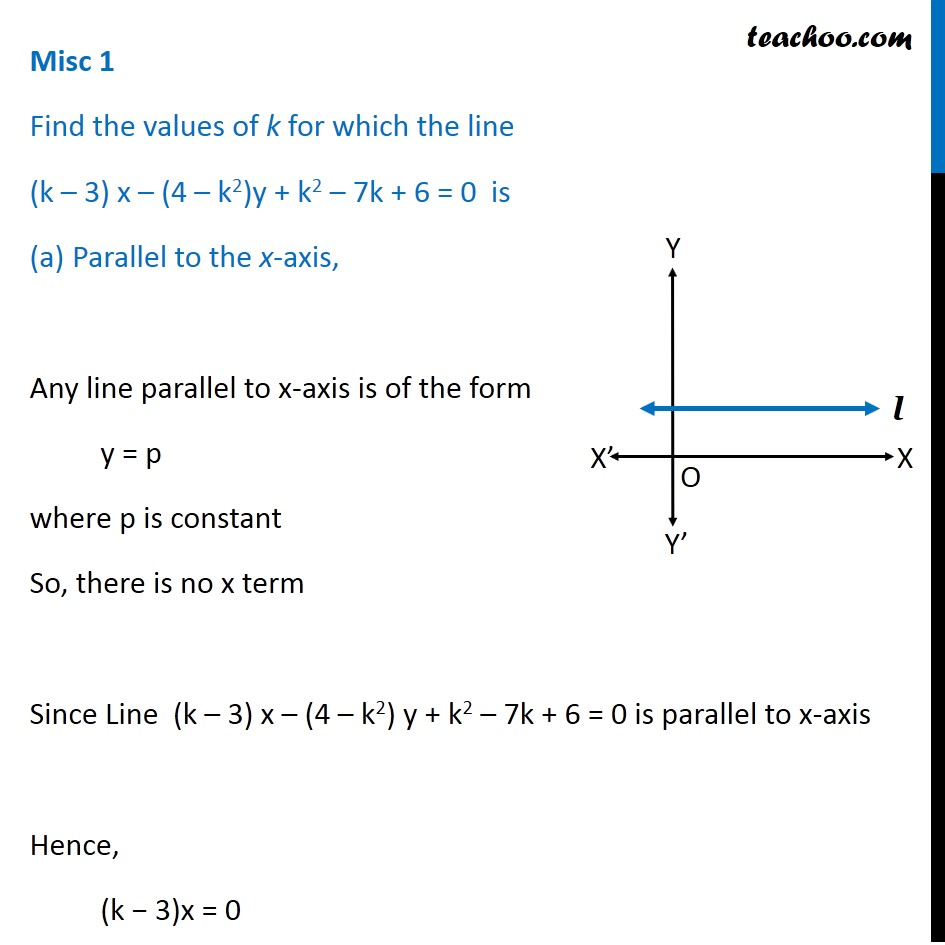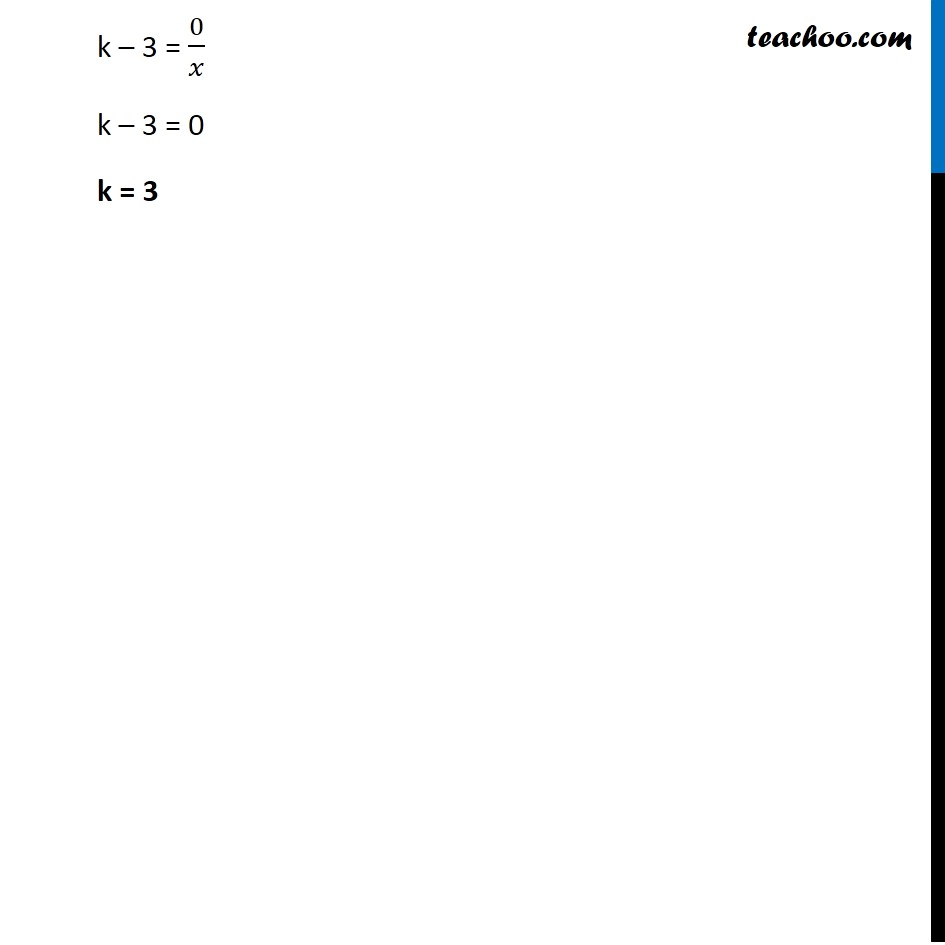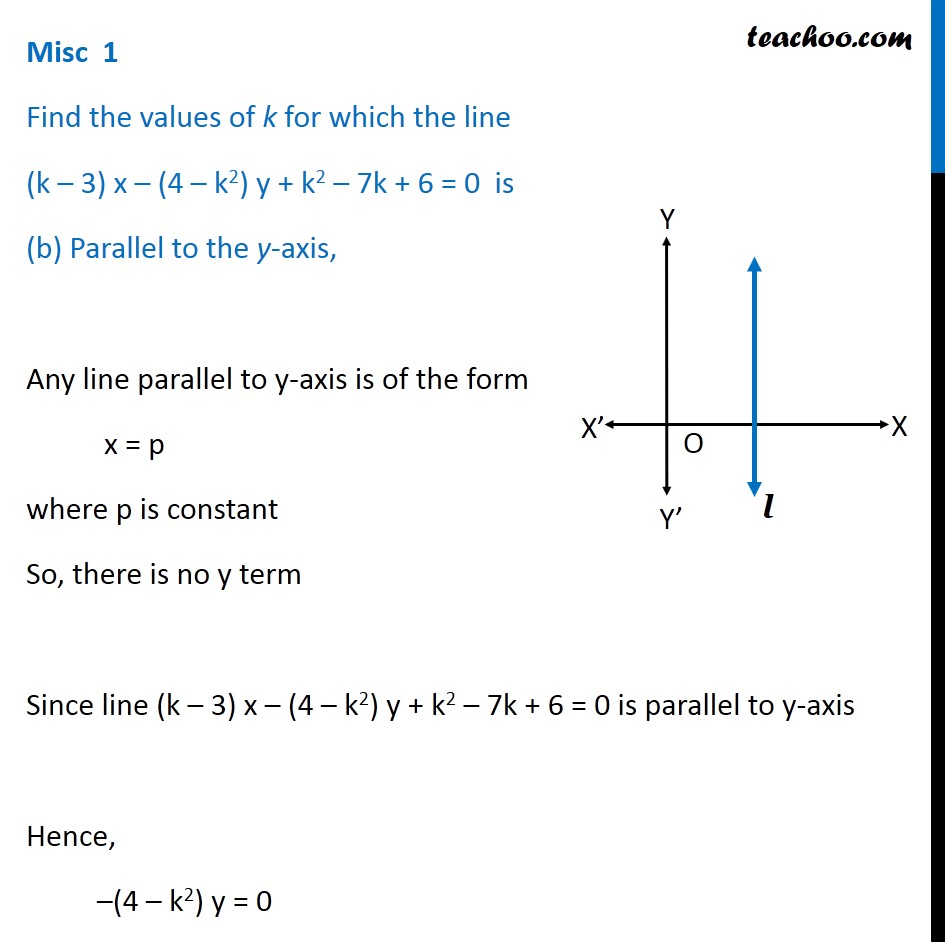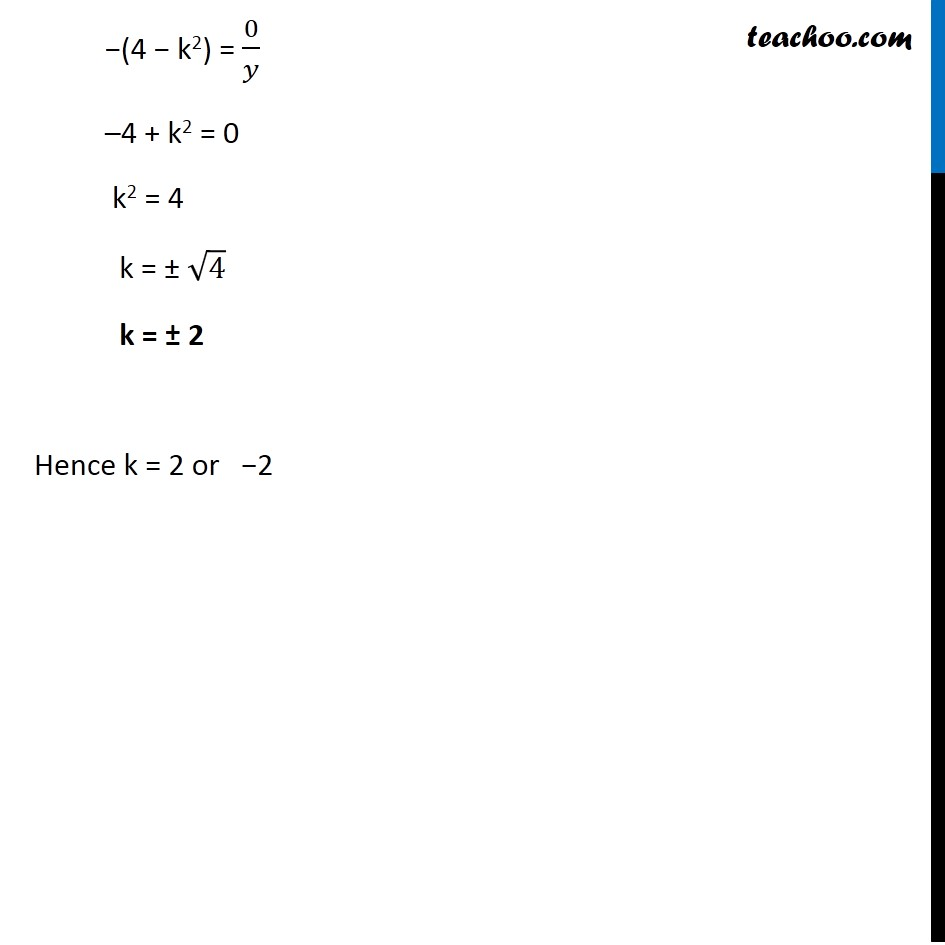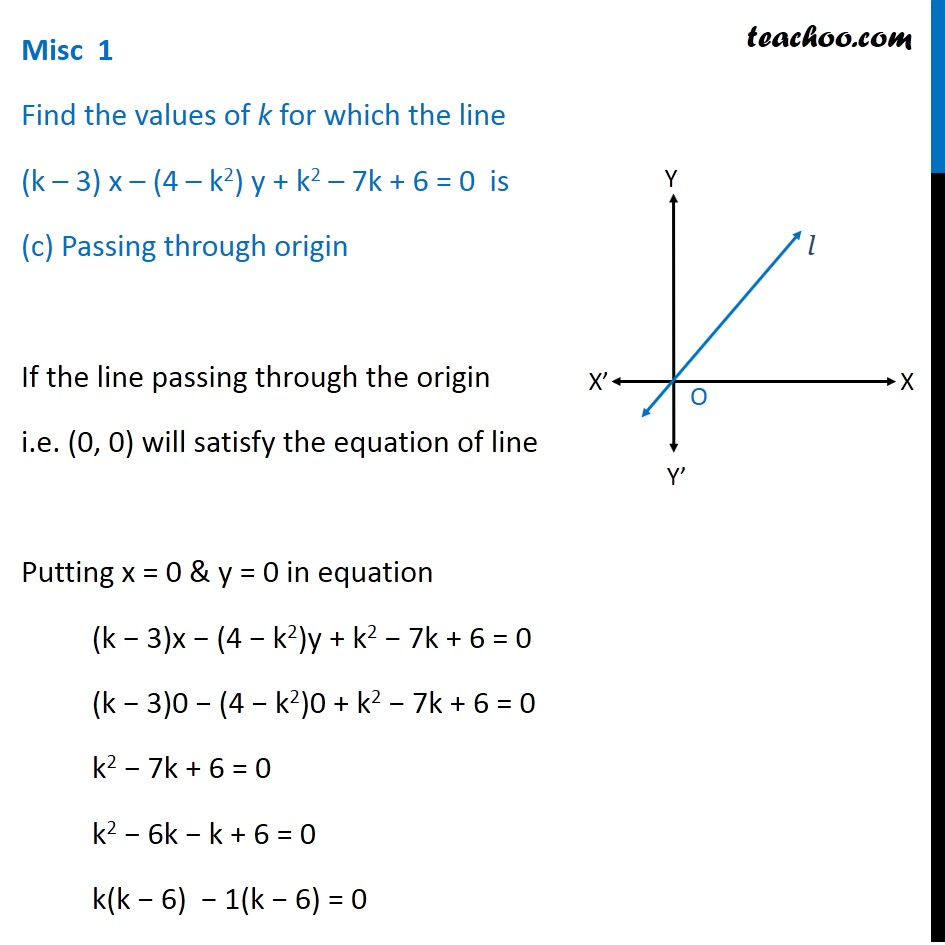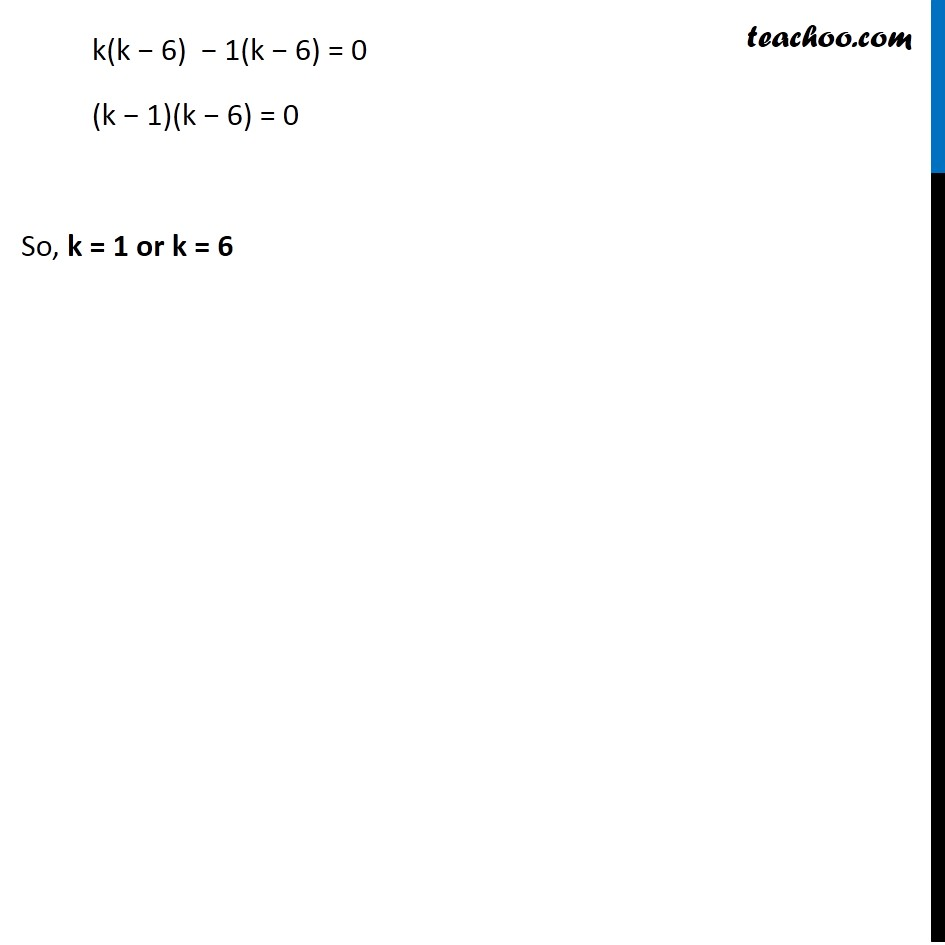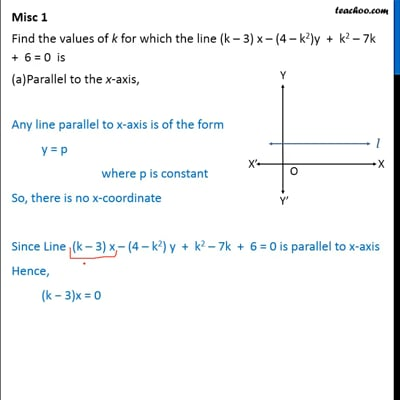This video is only available for Teachoo black users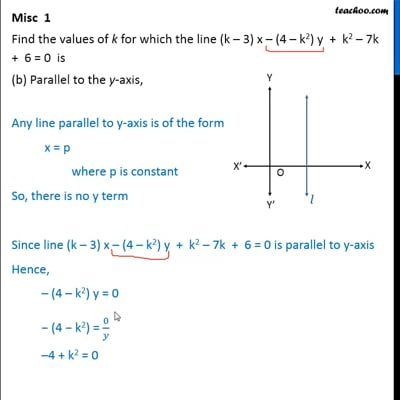This video is only available for Teachoo black users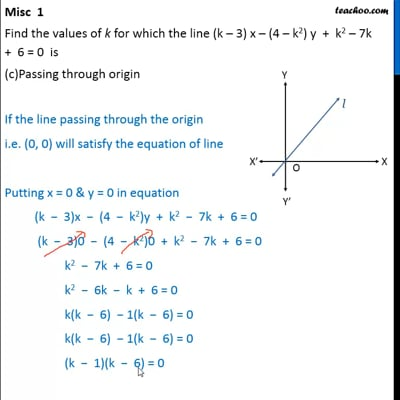This video is only available for Teachoo black users

### Transcript

Misc 1 Find the values of k for which the line (k – 3) x – (4 – k2)y + k2 – 7k + 6 = 0 is Parallel to the x-axis, Any line parallel to x-axis is of the form y = p where p is constant So, there is no x term Since Line (k – 3) x – (4 – k2) y + k2 – 7k + 6 = 0 is parallel to x-axis Hence, (k − 3)x = 0 k – 3 = 0/𝑥 k – 3 = 0 k = 3 Misc 1 Find the values of k for which the line (k – 3) x – (4 – k2) y + k2 – 7k + 6 = 0 is (b) Parallel to the y-axis, Any line parallel to y-axis is of the form x = p where p is constant So, there is no y term Since line (k – 3) x – (4 – k2) y + k2 – 7k + 6 = 0 is parallel to y-axis Hence, –(4 – k2) y = 0 −(4 − k2) = 0/𝑦 –4 + k2 = 0 k2 = 4 k = ± √4 k = ± 2 Hence k = 2 or −2 Misc 1 Find the values of k for which the line (k – 3) x – (4 – k2) y + k2 – 7k + 6 = 0 is (c) Passing through origin If the line passing through the origin i.e. (0, 0) will satisfy the equation of line Putting x = 0 & y = 0 in equation (k − 3)x − (4 − k2)y + k2 − 7k + 6 = 0 (k − 3)0 − (4 − k2)0 + k2 − 7k + 6 = 0 k2 − 7k + 6 = 0 k2 − 6k − k + 6 = 0 k(k − 6) − 1(k − 6) = 0 k(k − 6) − 1(k − 6) = 0 (k − 1)(k − 6) = 0 So, k = 1 or k = 6